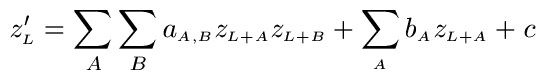### Problem Statement

Your task in this problem is to apply a simple quadratic filter to an image. We will define the intensity of pixel (i,j) as zi,j. To apply the filter we will find new intensities, z'i,j based on a quadratic formula:Here the a's are the coefficients for the quadratic terms, while the b's are the coefficients for the linear terms, and c is a constant. A, B and L all represent vectors with two components: an x component and a y component.

For instance, imagine that a(0,0),(0,0) = 1, while a(i,j),(i,j) = -0.25 when |i| + |j| = 1, and that b(0,0) = 1 while c = 0 and all other coefficients are 0. This corresponds to a filter that squares the intensity of each pixel, subtracts one quarter of the square of each of the four neighboring pixels, and finally adds the original value of the pixel. For instance,Your task is to compute the image after applying a specified filter. The filter will be randomly generated, but will have some structure to it.

The filter will be generated by first selecting K, the maximum offset in the x and y directions as an integer in [1,10]. This gives a total of (2*K+1)2 pixels that can be used in the filter terms. The constant term will be selected from a normal distribution with mean 0 and standard deviation 1. A subset of the (2*K+1)2 pixels will be chosen with size uniformly selected as J=[1,(2*K+1)2] (the size will be chosen first and then the subset). Only these offsets will be used in the terms of the filter. The coefficients for all the linear terms will be selected from a normal distribution with mean 0 and standard deviation 1. To generate the quadratic coefficients, the following process will be used. First, two vectors will be chosen, each with values corresponding to the J pixels selected and each selected from a normal distribution with mean 0 and standard deviation 1. The outer product of these two vectors will be taken to give the coefficients for all the quadratic terms. This process will be repeated J times to give J sets of coefficients for the quadratic terms. A weighted average of these J sets will be taken, where the weight of set i is pi = rand()*pi-1 and p0=1.

Your score will be based on the accuracy and runtime of your solution. While it is important that your return accurately applies the filter, small errors in favor of runtime will result in a higher overall score. More specifically, let MIN be the correct minimum intensity (over all color channels) and MAX be the correct maximum intensity. Your scaled error on each color channel of each pixel will be (YOUR INTENSITY - CORRECT INTENSITY) / (MAX - MIN). We will let E be the average over all pixels and channels of the square of this scaled error, and R be your runtime in milliseconds. Your score will be (NUMBER OF PIXELS) * J / (R + E * 1E8).

The input intensities will be given to you in row major order, where the intensity at row r, column c is given by element r*W+c of the input. The intensities for the three color channels will be given as three inputs.

b(dr,dc) is given by b[(dr + K) * (K+K+1) + (dc + K)].

a(dr1,dc1),(dr2,dc2) is given by a[((dr1 + K) * (K+K+1) + (dc1 + K)) * (K+K+1) * (K+K+1) + (dr2 + K) * (K+K+1) + (dc2 + K)]

Your return should be double precision, with 3 times as many elements as the input. If there are N pixels in the input, the first N values of your return should represent the new red values in the same order. The next N elements should represent the green values, while the final N elements should represent the new blue values.

A tool for testing the examples offline is provided.

### Definition

 Class: ImageFilter Method: filter Parameters: int, int, int, double[], double[], double[], double[], double[], double Returns: double[] Method signature: double[] filter(int W, int H, int K, double[] red, double[] green, double[] blue, double[] a, double[] b, double c) (be sure your method is public)

### Notes

-Treat areas outside the image as if they had value 0
-The time limit is 60 seconds, and the memory limit is 1GB.
-While the input intensities have all been scaled to be between 0 and 1, they were originally integers in [0,65535], which were divided by 65535 to obtain the scaled values you will use.
-The test images, like the examples will all be 1000x1000 pixels, and come from images with 16 bits per channel.
-The AMD ACML library has a number of matrix and signal processing function which you may find useful.
-You should treat the three color channels completely independently -- apply the filter to each one independently of the others.

### Examples

0)

 `"1 DSC_3695.png"`
```Returns: "K = 1<br>
J = 7<br>
"```
 View input or output.
1)

 `"2 DSC_3632.png"`
```Returns: "K = 2<br>
J = 16<br>
"```
 View input or output.
2)

 `"3 DSC_3571.2.png"`
```Returns: "K = 3<br>
J = 34<br>
"```
 View input or output.
3)

 `"4 DSC_3692.png"`
```Returns: "K = 4<br>
J = 46<br>
"```
 View input or output.
4)

 `"5 DSC_3104.png"`
```Returns: "K = 5<br>
J = 66<br>
"```
 View input or output.
5)

 `"6 DSC_2964.png"`
```Returns: "K = 6<br>
J = 144<br>
"```
 View input or output.
6)

 `"7 DSC_2913.png"`
```Returns: "K = 7<br>
J = 108<br>
"```
 View input or output.
7)

 `"8 DSC_3472.png"`
```Returns: "K = 8<br>
J = 229<br>
"```
 View input or output.
8)

 `"9 DSC_3460.png"`
```Returns: "K = 9<br>
J = 27<br>
"```
 View input or output.
9)

 `"10 DSC_3444.png"`
```Returns: "K = 10<br>
J = 95<br>
"```
 View input or output.

#### Problem url:

http://www.topcoder.com/stat?c=problem_statement&pm=10202

#### Problem stats url:

http://www.topcoder.com/tc?module=ProblemDetail&rd=13722&pm=10202